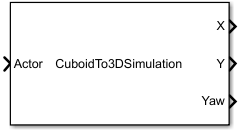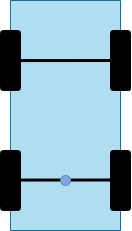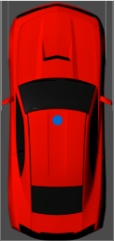Main Content

# Cuboid To 3D Simulation

Convert actor from cuboid coordinates to 3D simulation coordinates

• Library:
• Automated Driving Toolbox / Driving Scenario and Sensor Modeling

•## Description

The Cuboid To 3D Simulation block converts a cuboid actor pose in world coordinates to the X, Y, and Yaw coordinates used by the Simulation 3D Vehicle with Ground Following block. Use the converted values to set vehicle positions within the 3D simulation environment for actors created using the Driving Scenario Designer app. The ground terrain of the scene determines the roll (x-axis rotation), pitch (y-axis rotation), and elevation (z-axis position) of the vehicle.

You can specify a bus containing a single actor pose or multiple actor poses. By default, the block converts the pose of the first actor in the bus. To specify the actor whose pose you want to convert, specify the `ActorID` of that actor.

In cuboid and 3D simulation driving scenarios, the coordinate systems are the same, but the origins of vehicles differ. In cuboid driving scenarios, the vehicle origin is on the ground, under the center of the rear axle. The block transforms this origin to the origin used in the 3D simulation environment, which is under the geometric center of the vehicle. The table shows the origin difference between the two environments.

Cuboid Vehicle Origin3D Simulation Vehicle Origin## Ports

### Input

expand all

Cuboid actor pose in world coordinates, specified as a Simulink bus containing a MATLAB structure.

To obtain this structure input, use the Scenario Reader block to read actors from a scenario. By default, the Scenario Reader block outputs actors in ego vehicle coordinates. To convert these poses from ego vehicle to world coordinates, use the Vehicle To World block.

The structure in this bus can contain a single actor pose or multiple actor poses.

#### Single-Pose Structure

To specify a single actor pose, the structure must contain these fields.

FieldDescription
`ActorID`

Scenario-defined actor identifier, specified as a positive integer.

`Position`

Position of actor, specified as a real-valued vector of the form [x y z]. Units are in meters.

`Velocity`

Velocity (v) of actor in the x- y-, and z-directions, specified as a real-valued vector of the form [vx vy vz]. Units are in meters per second.

`Roll`

Roll angle of actor, specified as a real-valued scalar. Units are in degrees.

`Pitch`

Pitch angle of actor, specified as a real-valued scalar. Units are in degrees.

`Yaw`

Yaw angle of actor, specified as a real-valued scalar. Units are in degrees.

`AngularVelocity`

Angular velocity (ω) of actor in the x-, y-, and z-directions, specified as a real-valued vector of the form [ωx ωy ωz]. Units are in degrees per second.

#### Multiple-Pose Structure

To specify multiple actor poses, the structure must contain these fields.

FieldDescriptionType
`NumActors`Number of actorsNonnegative integer
`Time`Current simulation timeReal-valued scalar
`Actors`Actor poses`NumActors`-length array of actor pose structures

Each actor pose structure in `Actors` must have these fields.

FieldDescription
`ActorID`

Scenario-defined actor identifier, specified as a positive integer.

`Position`

Position of actor, specified as a real-valued vector of the form [x y z]. Units are in meters.

`Velocity`

Velocity (v) of actor in the x- y-, and z-directions, specified as a real-valued vector of the form [vx vy vz]. Units are in meters per second.

`Roll`

Roll angle of actor, specified as a real-valued scalar. Units are in degrees.

`Pitch`

Pitch angle of actor, specified as a real-valued scalar. Units are in degrees.

`Yaw`

Yaw angle of actor, specified as a real-valued scalar. Units are in degrees.

`AngularVelocity`

Angular velocity (ω) of actor in the x-, y-, and z-directions, specified as a real-valued vector of the form [ωx ωy ωz]. Units are in degrees per second.

The block converts only one pose from the `Actors` array. To specify which pose to convert, select Specify Actor ID, and then specify the `ActorID` of the actor by using the ActorID used for conversion parameter.

### Output

expand all

Longitudinal position of the actor in 3D simulation coordinates, returned as a numeric scalar. Units are in meters.

In this coordinate system, when looking in the positive direction of the X-axis, the positive Y-axis points left, and the Z-axis points up.

To specify the X-position of a vehicle in the 3D simulation environment, connect this port to the X input port of a Simulation 3D Vehicle with Ground Following block.

Lateral position of the actor in 3D simulation coordinates, returned as a numeric scalar. Units are in meters.

In this coordinate system, when looking in the positive direction of the X-axis, the positive Y-axis points left, and the Z-axis points up.

To specify the Y-position of a vehicle in the 3D simulation environment, connect this port to the Y input port of a Simulation 3D Vehicle with Ground Following block.

Yaw orientation angle of the actor about the Z-axis in 3D simulation coordinates, returned as a numeric scalar. Units are in degrees.

In this coordinate system, when looking in the positive direction of the Z-axis, yaw is clockwise-positive. However, if you view the simulation from a 2D top-down perspective, then yaw is counterclockwise-positive, because you are viewing the scene along the negative Z-axis.

To specify the yaw orientation angle of a vehicle in the 3D simulation environment, connect this port to the Yaw input port of a Simulation 3D Vehicle with Ground Following block.

## Parameters

expand all

Select this parameter to enable the ActorID used for conversion parameter, where you can specify the `ActorID` of the cuboid actor pose to convert to 3D simulation coordinates.

If you clear this parameter, then the block converts the first actor pose in the input Actor bus.

`ActorID` value of the cuboid actor to convert to 3D simulation coordinates, specified as a positive integer. This parameter must be a valid `ActorID` from the input Actor bus.

#### Dependencies

To enable this parameter, select Specify Actor ID.

• `Interpreted execution` — Simulate the model using the MATLAB interpreter. This option shortens startup time. In ```Interpreted execution``` mode, you can debug the source code of the block.

• `Code generation` — Simulate the model using generated C/C++ code. The first time you run a simulation, Simulink generates C/C++ code for the block. The C code is reused for subsequent simulations as long as the model does not change. This option requires additional startup time.

## See Also

Introduced in R2020a

## Support

#### Implementing an Adaptive Cruise Controller with Simulink

Download technical paper